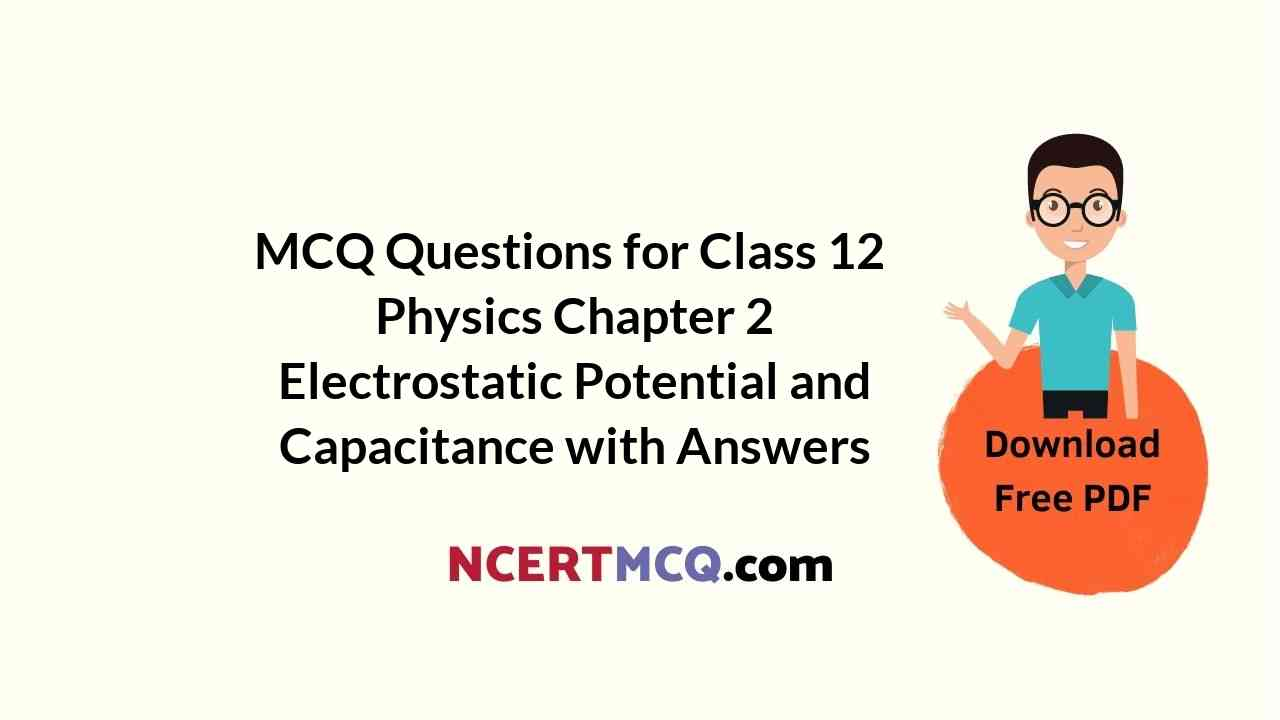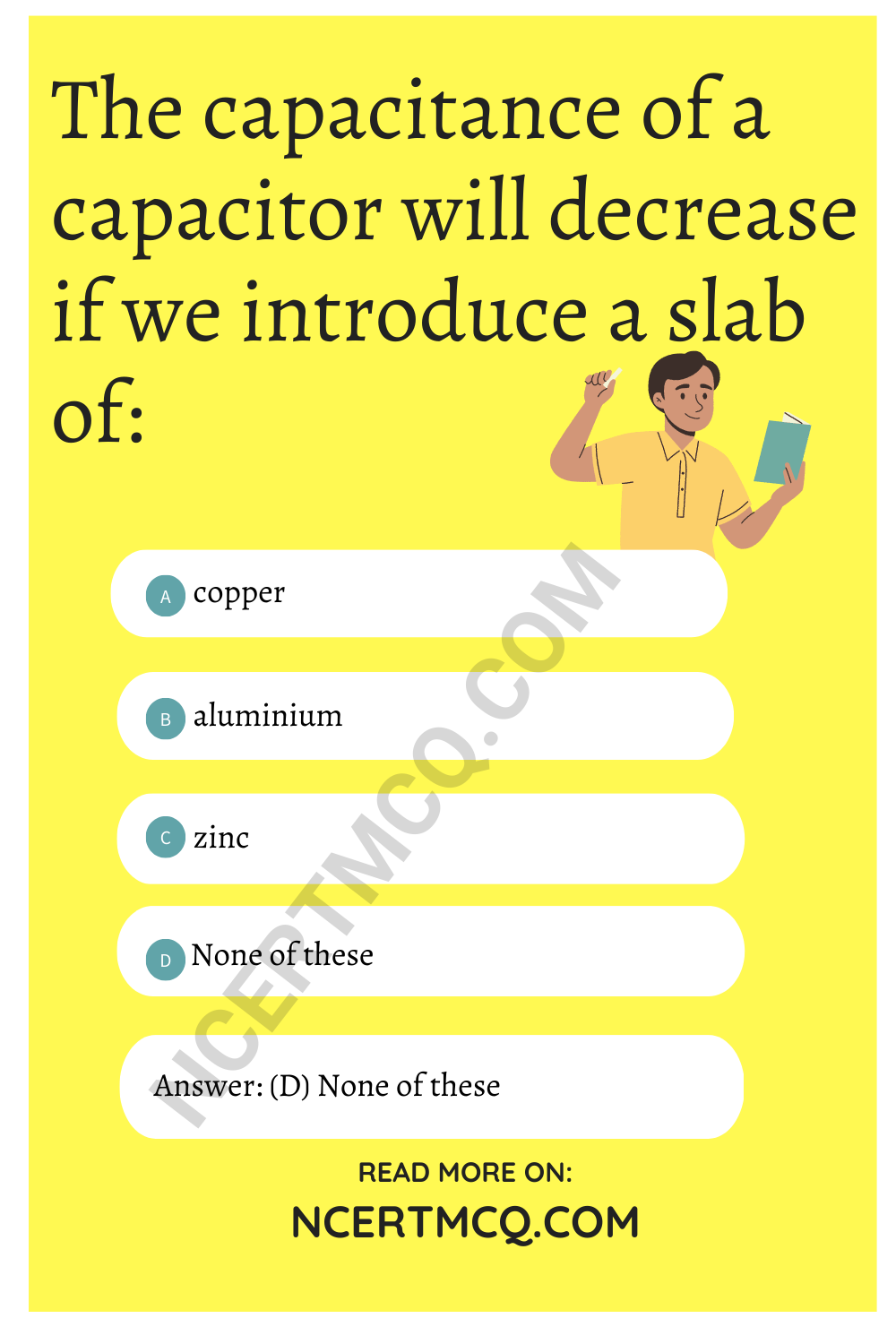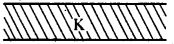Check the below Online Education NCERT MCQ Questions for Class 12 Physics Chapter 2 Electrostatic Potential and Capacitance with Answers Pdf free download. MCQ Questions for Class 12 Physics with Answers were prepared based on the latest exam pattern. We have provided Electrostatic Potential and Capacitance Class 12 Physics MCQs Questions with Answers to help students understand the concept very well.

## Electrostatic Potential and Capacitance Class 12 MCQs Questions with Answers

Class 12 Physics Chapter 2 MCQ Question 1.
The dimensions of fall of potential per unit distance are given by:
(a) [MLT-3 A-1]
(b) [ML2T-2A-1]
(c) [ML2T-2A-3]
(d) [MLT-2A-2]

Electrostatic Potential And Capacitance MCQ Question 2.
Which of the following is blocked by a capacitor ?
(a) A.C.
(b) D.C.
(c) Both A.C. and D.C.
(d) Neither A.C. nor D. C

Chapter 2 Physics Class 12 MCQ Question 3.
Two copper spheres of the same radius, one solid and the other hollow, are charged to the same potential. Which will have more charge?
(a) Solid sphere
(b) Hollow sphere
(c) Both will have an equal charge
(d) None of these

Answer: (c) Both will have an equal charge

Electrostatic Potential And Capacitance Class 12 MCQ Question 4.
The capacitance of a capacitor will decrease if we introduce a slab of:
(a) copper
(b) aluminium
(c) zinc
(d) None of theseElectric Potential And Capacitance MCQ Question 5.
Two capacitors of capacitance 6 µF and 4 µF are put in series across a 120 V battery. What is the potential difference across the 4 µF capacitor ?
(a) 72 V
(b) 60 V
(c) 48 V
(d) zero

Physics Class 12 Chapter 2 MCQ Question 6.
In which of the following forms is the energy stored in a capacitor?
(a) Charge
(b) Potential
(c) Capacitance
(d) Electric field

Ch 2 Physics Class 12 MCQ Question 7.
Two conducting spheres of radii r1 and r2 are equally charged. The ratio of their potential is
(a) (r1/r2
(b) (r1r2
(c)(r1/r2)
(d) (r2/r1)

MCQ On Electrostatic Potential And Capacitance Question 8.
Twenty seven drops of mercury are charged simultaneously to the same potential of 10 volts. What will be potential if all the charged drops are made to combine to form one large drop ?
(a) 180 V
(b) 90 V
(c) 120 V
(d) 45 V

MCQ Of Chapter 2 Physics Class 12 Question 9.
The P.E. of an electric dipole is maximum when it makes an angle θ with electric field. The value of θ is :
(a) $$\frac{π}{2}$$
(b) π
(c) zero
(d) $$\frac{3π}{2}$$

MCQ Of Electrostatics Class 12 Question 10.
The amount of work required to increase the distance between -6µC and 4µC from 6 cm to 18 cm will be :
(a) 1.8 J
(b) 2.4 J
(c) 1.8 µJ
(d) 2.4 µJ

Class 12 Physics Ch 2 MCQ Question 11.
An electron initially at rest is accelerated through a potential difference of one volt. The energy gained by electron is:
(a) 1 J
(b) 1.6 × 10-19 J
(c) 10-19 J
(d) None of these

Answer: (b) 1.6 × 10-19 J

Physics Chapter 2 MCQ Class 12 Question 12.
A bird sitting on a high power line:
(a) gets killed instantly
(b) gets a mild shock
(c) gets a fatal shock
(d) is not affected practically

Answer: (d) is not affected practically

MCQ On Electrostatics Class 12 Question 13.
Choose the SI unit of electric potential energy :
(a) Joule
(b) Coulomb
(c) Netwon per coulomb
(d) Erg

Electrostatics Class 12 MCQ Question 14.
The capacitance of earth, viewed as a spherical conductor of radius 6408 km is:
(a) 1420 µF
(b) 712 µF
(c) 680 µF
(d) 540 µF

MCQs Of Physics Class 12 Chapter 2 Question 15.
A capacitor is connected to a cell of emf E having some internal resistance r, the potential across the:
(a) cell is < E
(b) cell is E
(b) cell is > E
(d) capacitor is E

Class 12 Physics Chapter 2 MCQ Questions And Answers Question 16.
If a +ve charge is moved from low to high potential region,
the electric potential energy:
(a) decreases
(b) increases
(c) remain the same
(d) may increase or decrease

MCQ Class 12 Physics Chapter 2 Question 17.
A capacitor is connected across a battery and the plate separation of capacitor is increased without removing the battery, then:
(a) capacitance will increase
(b) charge stored will increase
(c) energy stored will decrease
(d) potential difference will increase

Answer: (c) energy stored will decrease

MCQ On Capacitors Class 12 Question 18.
Two capacitors of 3 µF and 6 µF are connected in series with a battery of P.d. 12 V. The P.d. across 3µF and 6µF
capacitors respectively will be :
(a) 8 V, 4 V
(b) 6 V, 6 V
(c) 4 V, 8 V
(d) 9 V, 3 V

Answer: (a) 8 V, 4 V

MCQ Questions For Class 12 Physics Chapter 2 Question 19.
An electric charge of 425 f µC is removed from a fully charged capacitor of capacitance 8.5µF. Its potential will be lowered by:
(a) 75 V
(b) 100 V
(c) 85 V
(d) 50 V

Question 20.
A dielectric is placed in between the two parallel plates of a capacitor as shown in the figure. The dielectric constant of the dielectric being K. If the initial capacity is C, then the new capacity will be:(a) (K + l).C
(b) K.C
(c) $$\frac{(K+1)}{2}$$ C
(d) (k – l) C

Answer: (c) $$\frac{(K+1)}{2}$$ C

Question 21.
Minimum number of capacitor of 2µF each required to obtain a capacitance of 5µF will be:
(a) 4
(b) 3
(c) 5
(d) 6

Question 22.
When a dielectric slab is introduced between the plates of a parallel plate capacitor which remains connected to a battery then charge on the plates relative to earlier charge is :
(a) more
(b) less
(c) less or may be more
(d) same

Ans. (a)
Question 23.
Choose the vector physical quantity:
(a) Electric flux
(b) Electric potential
(c) Electric potential energy
(d) Electric intensity

Question 24.
The surface density of charge is measure:
(a) Cm-1
(b) Cm-2
(c) Cm-3
(d) JC-1

Question 25.
A polythene piece rubbed with wool is found to have a negative charge of 6.4 × 10-9c. How many electrons have been transferred during the process?
(a) 4 × 1012
(b) 4 × 1011
(c) 4 × 1010
(d) None of these

Question 26.
In the above question:
(a) no electrons are transferred
(b) electrons are transferred from wool to x polythene
(c) electrons are transferred from polythene to wool
(d) nothing can be decided

Answer: (b) electrons are transferred from wool to x polytheneQuestion 27.
In previous question, mass transferred to polythene is:
(a) 3.64 × 1020 kg
(b) 4 × 10-28 kg
(c) 4 × 1010 kg
(d) None of these

Answer: (a) 3.64 × 1020 kg

Question 28.
What is the number of electrons in 100 g water?
(a) 3.35 × 1024
(b) 3.35 × 1025
(c) 6.02 × 1023
(d) None of these

Question 29.
A uniform electric field of 10 NC-1 exists in the vertically downward direction. What is the increase in the electric potential asonegoes up through a height of 300 cm ?
(a) 15 V
(b) 30 V
(c) 45V
(d) None of these

Question 30.
The electric potential of earth is taken as:
(a) zero
(b) infinity
(c) unity
(d) None of these